# Identify the odd one out using direction, position and number

In this worksheet, students will identify an image that does not fit in with the rest of the group. The focus will be on direction, position and number, so students will need to look at where shapes are, which direction they are facing, and how many there are.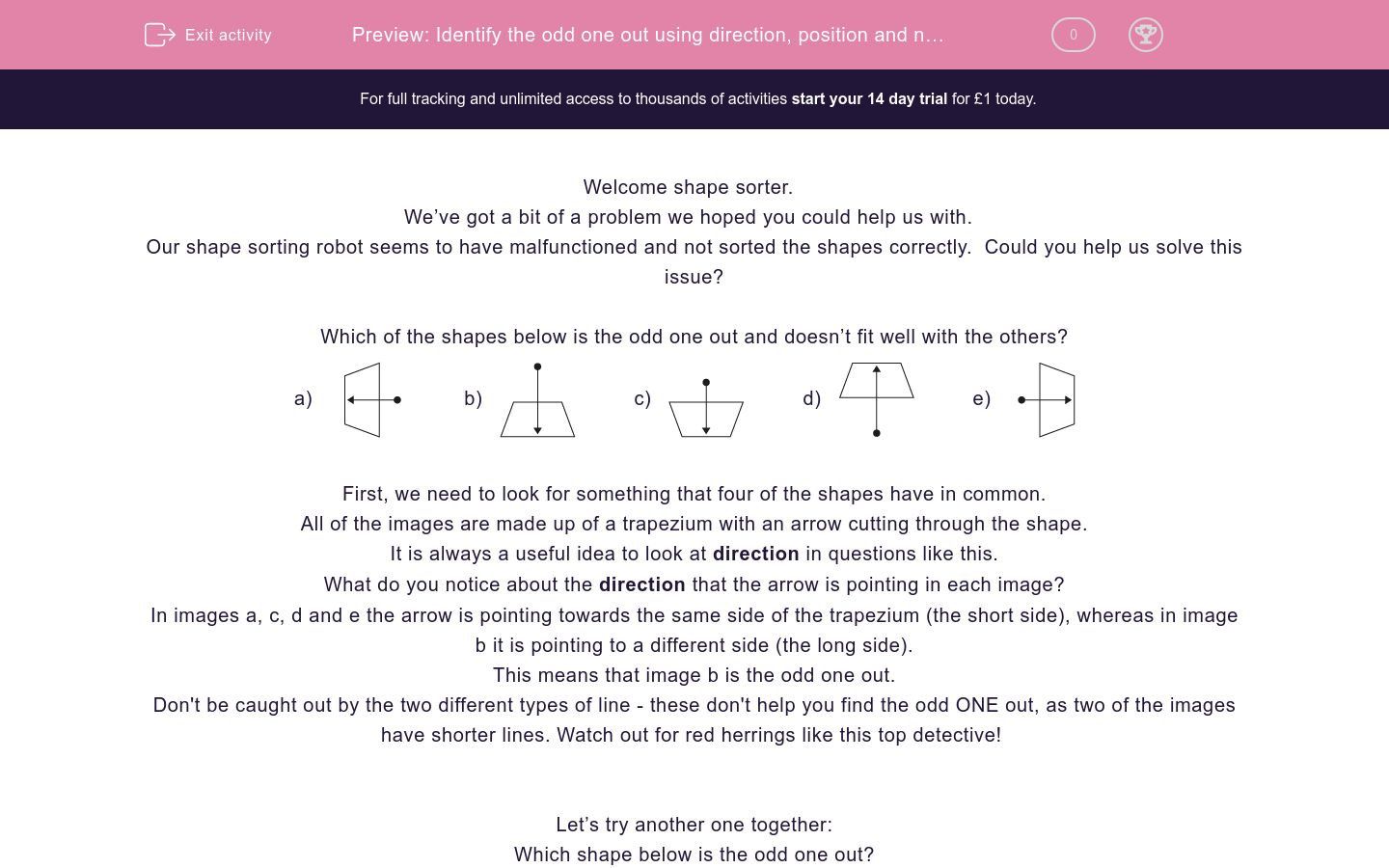Key stage:  KS 2

Curriculum topic:  Spatial and Non-Verbal Reasoning

Curriculum subtopic:  Odd One Out

Difficulty level:### QUESTION 1 of 10

Welcome shape sorter.

We’ve got a bit of a problem we hoped you could help us with.

Our shape sorting robot seems to have malfunctioned and not sorted the shapes correctly.  Could you help us solve this issue?

Which of the shapes below is the odd one out and doesn’t fit well with the others?

a)b)c)d)e)First, we need to look for something that four of the shapes have in common.

All of the images are made up of a trapezium with an arrow cutting through the shape.

It is always a useful idea to look at direction in questions like this.

What do you notice about the direction that the arrow is pointing in each image?

In images a, c, d and e the arrow is pointing towards the same side of the trapezium (the short side), whereas in image b it is pointing to a different side (the long side).

This means that image b is the odd one out.

Don't be caught out by the two different types of line - these don't help you find the odd ONE out, as two of the images have shorter lines. Watch out for red herrings like this top detective!

Let’s try another one together:

Which shape below is the odd one out?

a)b)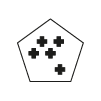c)d)e)First, we need to look for something that four of the shapes have in common.

In questions like this, counting is really important. What could we count?

We have two things to count here: the number of mini shapes and the number of sides of the main shape.

Image a is made up of a 3-sided shape with 3 crosses inside.

Image b is a 5-sided shape with 5 crosses inside.

Image c is a 4-sided shape with 4 crosses inside.

Image d is a 6-sided shape with 5 crosses inside.

Image e is a 4-sided shape with 4 crosses inside.

This means that image d is the odd one out as the number of sides of the main shape does not match the number of mini shapes inside, unlike the other options.

It’s now your turn to identify the odd image.

Remember to look at the direction and position of shapes within the image and always see if there is something you can count.

Good luck shape sorter!

Which of the images below is the odd one out?

a)b)c)d)e)Which of the images below is the odd one out?

a)b)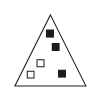c)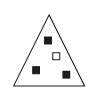d)e)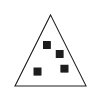Which of the images below is the odd one out?

a)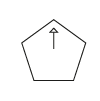b)c)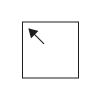d)e)Which of the images below is the odd one out?

a)b)c)d)e)Which of the images below is the odd one out?

a)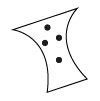b)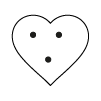c)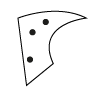d)e)Which of the images below is the odd one out?

a)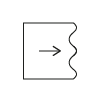b)c)d)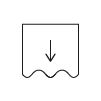e)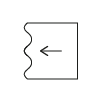Which of the images below is the odd one out?

a)b)c)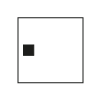d)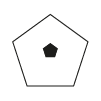e)Which of the images below is the odd one out?

a)b)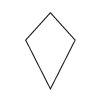c)d)e)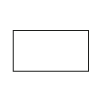Which of the images below is the odd one out?

a)b)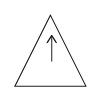c)d)e)Which of the images below is the odd one out?

a)b)c)d)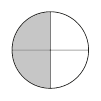e)• Question 1

Which of the images below is the odd one out?

e)EDDIE SAYS
This question involves positioning and layering. The most important element is the position of the black rectangle. In options a, b, c and d the black rectangle is positioned underneath the white rectangle. The black rectangle isn't underneath the white rectangle in option e, so it is the odd one out.
• Question 2

Which of the images below is the odd one out?

b)EDDIE SAYS
All the outer shapes are triangles which doesn't help us, so we know that we need to look inside to find the odd one out. We have a choice on what we could count here. We could choose the number of white squares, the number of black squares or the total number of squares. If we count how many squares there are in total in each triangle, we can see that options a, c, d and e have a total of 4 squares inside each. Option b has 5 squares, making it the odd one out.
• Question 3

Which of the images below is the odd one out?

d)EDDIE SAYS
It's time for another direction question now! This time it is more challenging as all of the shapes and the arrows look different. Get your detective's magnifying glass out and look closely at where the arrow is pointing in each shape. What can you see? The arrows in options a, b, c and e are pointing to a corner. The silly arrow in option d is pointing to a side rather than a corner, which makes it the odd one out.
• Question 4

Which of the images below is the odd one out?

d)EDDIE SAYS
This question is counting-themed. Each rectangle is split up into five equal pieces, with some pieces shaded and some decorated. In options a, b, c and e there are a total of three segments either shaded or decorated. Option d has an extra segment shaded, making it the odd one out.
• Question 5

Which of the images below is the odd one out?

a)EDDIE SAYS
The outer shapes are all different so this is a red herring and won't help us reach the correct answer. I hope you kept your counting hat on! Options b, c, d and e all have 3 dots inside them, whereas option a has 4. This means that option a is the odd one out.
• Question 6

Which of the images below is the odd one out?

b)EDDIE SAYS
This question focuses on direction. In options a, c, d and e the arrow is pointing in the direction of the wiggly edge. In option b, the arrow is pointing towards a straight edge instead so this is the odd on out.
• Question 7

Which of the images below is the odd one out?

d)EDDIE SAYS
This one is another position-themed question! In options a, b, c and e there is a black inner shape on the left-hand side of the outer shape. In option d the black inner shape is the middle instead. This makes option d the odd one out? Can you name the shape shown in option d?
• Question 8

Which of the images below is the odd one out?

d)EDDIE SAYS
This is a counting-themed question. All the shapes shown in options a, b, c and e are quadrilaterals, so they have 4 sides. The shape shown in option d is a pentagon and has 5 sides - how greedy! This makes option d the odd one out.
• Question 9

Which of the images below is the odd one out?

a)EDDIE SAYS
This is a direction-focused question. Think carefully about where the image is pointing in each triangle. In options b, c, d and e the arrow is pointing to an inside corner or angle of each triangle. In option a the arrow is pointing towards a straight edge instead. This makes option a the odd one out.
• Question 10

Which of the images below is the odd one out?

c)EDDIE SAYS
It's time to count again in this clue detective! All of the circles are split into four equal parts (quarters) with some segments shaded. In options a, b, d and e there are 2 segments shaded, but in option c there are 3. This makes option c the odd one out for being an extra sneaky shader! Well done of spotting all those clues detective and recognising whether you needed to count, or focus on direction or positions.
---- OR ----

Sign up for a £1 trial so you can track and measure your child's progress on this activity.

### What is EdPlace?

We're your National Curriculum aligned online education content provider helping each child succeed in English, maths and science from year 1 to GCSE. With an EdPlace account you’ll be able to track and measure progress, helping each child achieve their best. We build confidence and attainment by personalising each child’s learning at a level that suits them.

Get startedStart your £1 trial today.
Subscribe from £10/month.